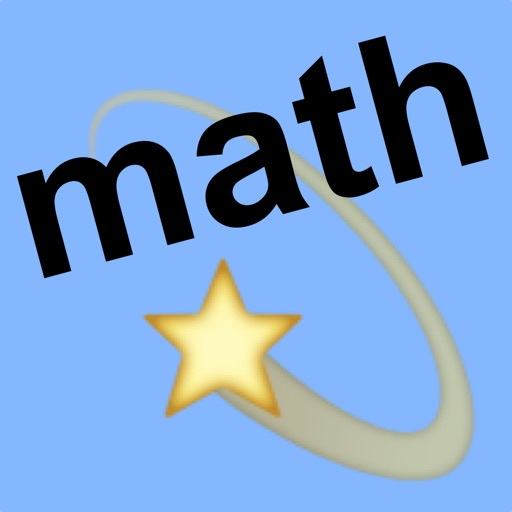## mathflair is a powerful learning aid to help students master rationalization of radicals, rational fractions, radical simplification, quadratic expressions and equations, simultaneous equations and much more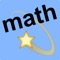# school math with flair

by Walter Kissach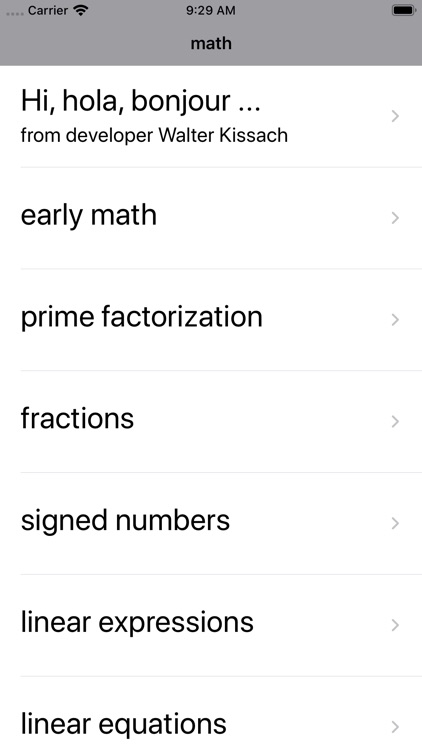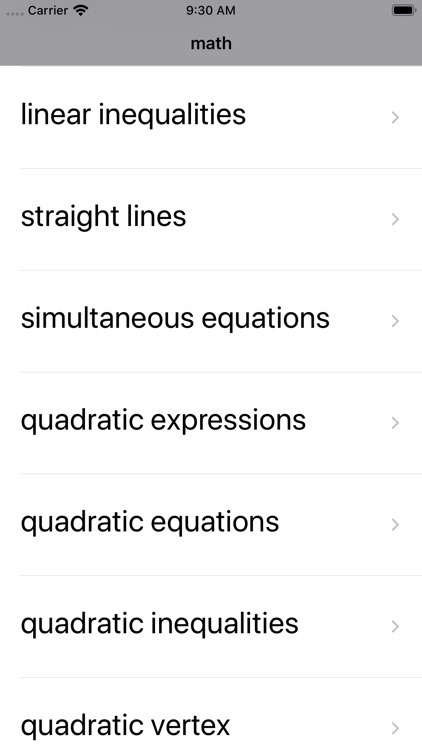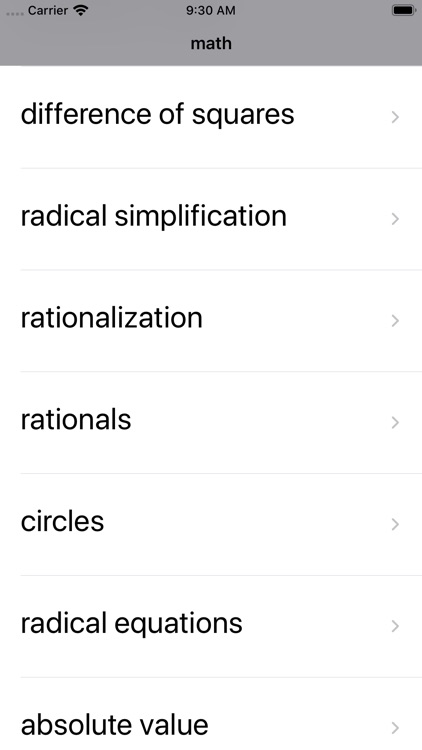mathflair is a powerful learning aid to help students master rationalization of radicals, rational fractions, radical simplification, quadratic expressions and equations, simultaneous equations and much more.### App Details

Version
7.0
Rating
NA
Size
13Mb
Genre
Education Reference
Last updated
January 22, 2020
Release date
March 27, 2012

### App Screenshots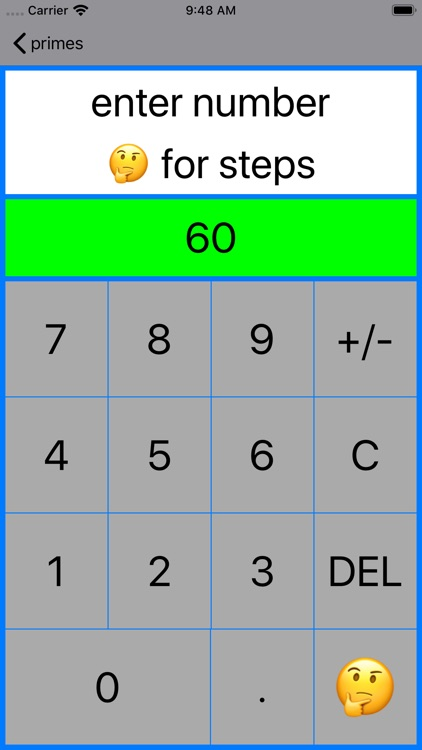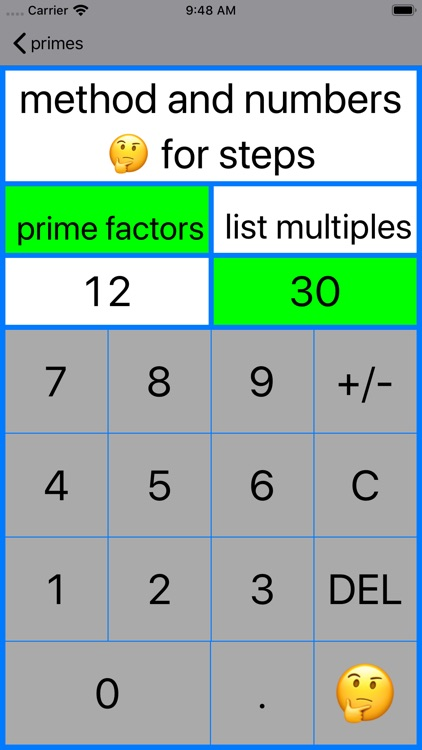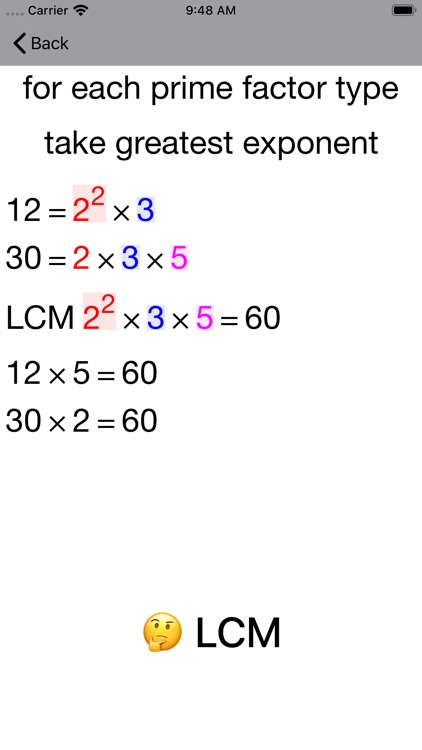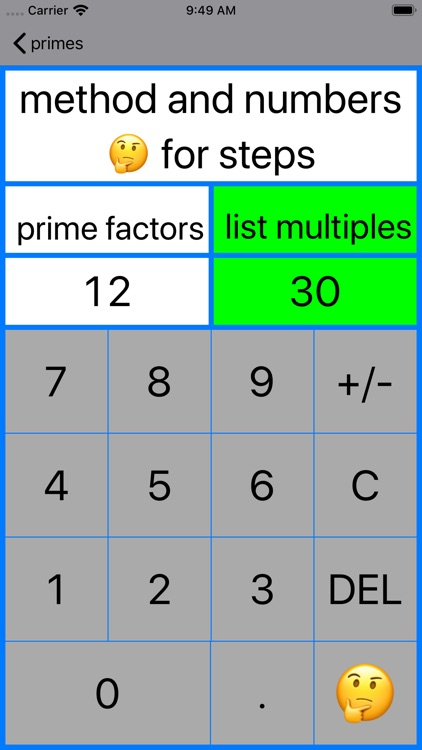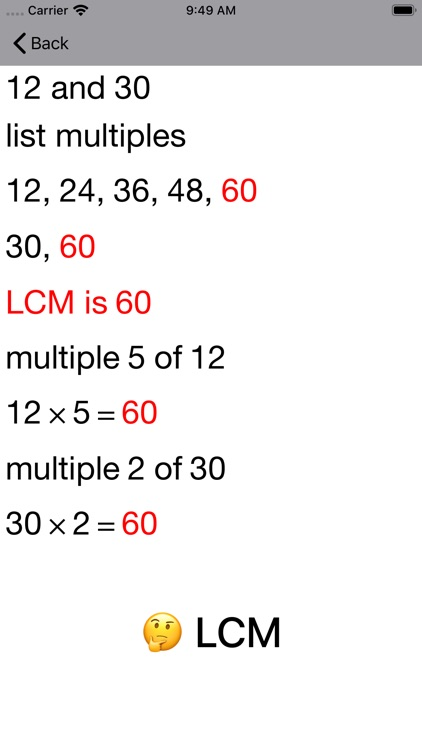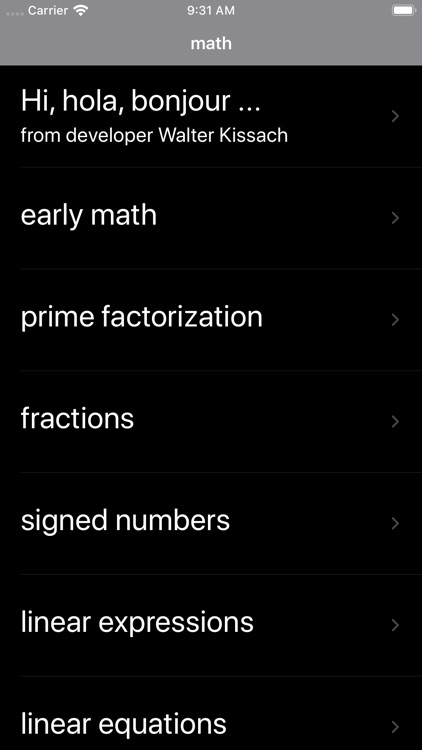### App Store Description

mathflair is a powerful learning aid to help students master rationalization of radicals, rational fractions, radical simplification, quadratic expressions and equations, simultaneous equations and much more.

Interactive Coaching Calculators and Guides combine to speed the learning process.

Modern NUMBER pencil and paper methods :

- Addition with Partial Sum and Column
- Subtraction with Trade First, Count Down and Partial Difference
- Multiplication with Partial Product, Lattice and Grid/Box
- Division with Partial Quotient
- Standard algorithms

Modern FRACTION pencil and paper methods :

- Addition and subtraction in stacked or line format
- Multiplication
- Division

PRIMES

- Prime factorization by factor tree

- LCM by prime factorization
- LCM by listing multiples

- GCD by prime factorization
- GCD (GCF) by listing divisors

SIGNED NUMBERS

- addition, subtraction of two signed numbers
- multiplication, division of two signed numbers

SOLVING LINEAR EQUATIONS

- one-step
x − 2 = 3, 5 + x = 4, 3x = -12, x/7 = 2

- two-step
3x + 8 = 20, x/9 + 2 = 5

- multi-step
4 + 9x = 2x + 18

SOLVING SIMULTANEOUS LINEAR EQUATIONS

- elimination method
- substitution method

SOLVING LINEAR INEQUALITIES

- one-step
x − 2 ≤ 3, 5 + x ≥ 4, 3x ≤ -12, x/7 ≥ 2

- two-step
3x + 8 ≥ 20, x/9 + 2 ≤ 5

- multi-step
4 + 9x ≥ 2x + 18

- by Difference of Squares
- by Guess and Check method
- by Grid or Box method
- by Grouping or AC method

- by factorization
- by completing the square

- by sketching associated graph

- by completing the square

- by expressing equation in centre-radius form

SIMPLIFYING SIMPLE RATIONAL FRACTIONS

- by factorization

SIMPLIFYING SQUARE ROOTS

- by perfect squares, factor trees and prime factorization

SIMPLIFYING HIGHER ROOTS

- up to sixth roots by prime factorization

- 2/√50, 6/(8 + √20), 4/(2√5 + 3√45)

SOLVING ABSOLUTE VALUE EQUATIONS AND INEQUALITIES

- |3x − 2| = 6, |2x − 3| ≥ 5